# PSAT Math : Triangles

## Example Questions

### Example Question #5 : How To Find The Length Of The Hypotenuse Of A Right Triangle : Pythagorean Theorem

A right triangle has side lengths of 21 and 72. What is the length of the hypotenuse?

75

67

84

80

70

75

Explanation:

By the Pythagorean Theorem, 212 + 722 = hyp2. Then hyp2 = 5625, and the hypotenuse = 75. If you didn't know how to figure out that 752 = 5625, that's okay. Look at the answer choices. We could easily have squared them and chosen the answer choice that, when squared, equals 5625.

### Example Question #1 : How To Find The Length Of The Hypotenuse Of A Right Triangle : Pythagorean Theorem

Sam and John both start at the same point.  Sam walks 30 feet north while John walks 40 feet west.  How far apart are they at their new locations?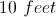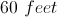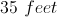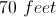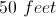Explanation:

Sam and John have walked at right angles to each other, so the distance between them is the hypotenuse of a triangle.  The distance can be found using the Pythagorean Theorem.

### Example Question #1 : How To Find The Length Of The Hypotenuse Of A Right Triangle : Pythagorean Theorem

Daria and Ashley start at the same spot and walk their two dogs to the park, taking different routes. Daria walks 1 mile north and then 1 mile east. Ashley walks her dog on a path going northeast that leads directly to the park. How much further does Daria walk than Ashley?

1 mile

√2 miles

2 – √2 miles

2 + √2 miles

Cannot be determined

2 – √2 miles

Explanation:

First let's calculate how far Daria walks. This is simply 1 mile north + 1 mile east = 2 miles. Now let's calculate how far Ashley walks. We can think of this problem using a right triangle. The two legs of the triangle are the 1 mile north and 1 mile east, and Ashley's distance is the diagonal. Using the Pythagorean Theorem we calculate the diagonal as √(12 + 12) = √2. So Daria walked 2 miles, and Ashley walked √2 miles. Therefore the difference is simply 2 – √2 miles.

### Example Question #1 : How To Find The Length Of The Hypotenuse Of A Right Triangle : Pythagorean Theorem

Which of the following sets of sides cannnot belong to a right triangle?

5, 12, 13

6, 7, 8

2, 2, 2√2

3, 4 ,5

2, 2√3, 4

6, 7, 8

Explanation:

To answer this question without plugging all five answer choices in to the Pythagorean Theorem (which takes too long on the GRE), we can use special triangle formulas. Remember that 45-45-90 triangles have lengths of x, x, x√2.  Similarly, 30-60-90 triangles have lengths x, x√3, 2x.  We should also recall that 3,4,5 and 5,12,13 are special right triangles.  Therefore the set of sides that doesn't fit any of these rules is 6, 7, 8.

### Example Question #11 : How To Find The Length Of The Hypotenuse Of A Right Triangle : Pythagorean Theorem

Max starts at Point A and travels 6 miles north to Point B and then 4 miles east to Point C. What is the shortest distance from Point A to Point C?

7 miles

4√2 miles

2√13 miles

5 miles

10 miles

2√13 miles

Explanation:

This can be solved with the Pythagorean Theorem.

62 + 42 = c2

52 = c2

c = √52 = 2√13

### Example Question #11 : How To Find The Length Of The Hypotenuse Of A Right Triangle : Pythagorean Theorem

Which set of side lengths CANNOT correspond to a right triangle?

6, 8, 11

8, 15, 17

7, 24, 25

3, 4, 5

5, 12, 13

6, 8, 11

Explanation:

Because we are told this is a right triangle, we can use the Pythagorean Theorem, a2 + b2 = c2. You may also remember some of these as special right triangles that are good to memorize, such as 3, 4, 5.

Here, 6, 8, 11 will not be the sides to a right triangle because 62 + 82 = 102.

### Example Question #12 : How To Find The Length Of The Hypotenuse Of A Right Triangle : Pythagorean Theorem

Angela drives 30 miles north and then 40 miles east. How far is she from where she began?

50 miles

60 miles

45 miles

35 miles

50 miles

Explanation:

By drawing Angela’s route, we can connect her end point and her start point with a straight line and will then have a right triangle. The Pythagorean theorem can be used to solve for how far she is from the starting point: a2+b2=c2, 302+402=c2, c=50. It can also be noted that Angela’s route represents a multiple of the 3-4-5 Pythagorean triple.

### Example Question #12 : How To Find The Length Of The Hypotenuse Of A Right Triangle : Pythagorean Theorem

To get from his house to the hardware store, Bob must drive 3 miles to the east and then 4 miles to the north. If Bob was able to drive along a straight line directly connecting his house to the store, how far would he have to travel then?

15 miles
25 miles
7 miles
9 miles
5 miles
Explanation:

Since east and north directions are perpendicular, the possible routes Bob can take can be represented by a right triangle with sides a and b of length 3 miles and 5 miles, respectively. The hypotenuse c represents the straight line connecting his house to the store, and its length can be found using the Pythagorean theorem: c2 = 32+ 42 = 25. Since the square root of 25 is 5, the length of the hypotenuse is 5 miles.

### Example Question #91 : Geometry

A park is designed to fit within the confines of a triangular lot in the middle of a city.  The side that borders Elm street is 15 feet long. The side that borders Broad street is 23 feet long. Elm street and Broad street meet at a right angle. The third side of the park borders Popeye street, what is the length of the side of the park that borders Popeye street?

22.5 feet

17.44 feet

27.46 feet

16.05 feet

18.5 feet

27.46 feet

Explanation:

This question requires the use of Pythagorean Theorem. We are given the length of two sides of a triangle and asked to find the third. We are told that the two sides we are given meet at a right angle, this means that the missing side is the hypotenuse. So we use a+ b= c2, plugging in the two known lengths for a and b. This yields an answer of 27.46 feet.

### Example Question #161 : Plane Geometry

A right triangle has legs of 15m and 20m. What is the length of the hypotenuse?

35m

40m

30m

45m

25m